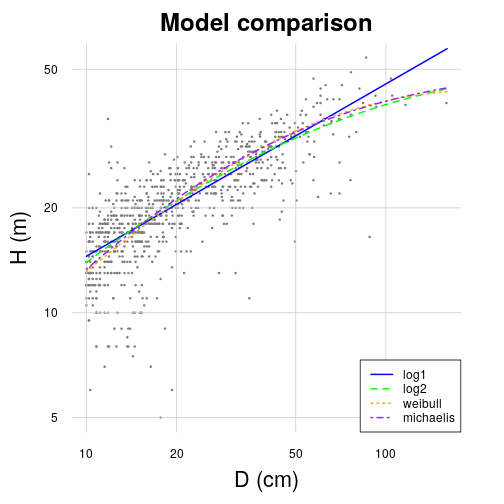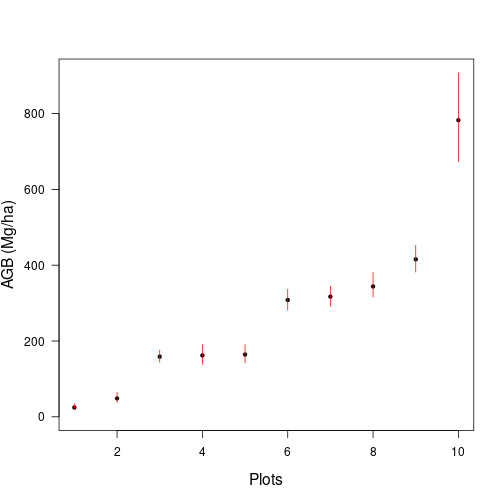#Load BIOMASS and datasets Install BIOMASS (to be done once)

``````install.packages("BIOMASS")
``````

``````require(BIOMASS)
require(knitr) # To build tables in this document
``````

Load the two datasets stored in the package

``````data(KarnatakaForest)
str(KarnatakaForest)
``````
``````## 'data.frame':    65889 obs. of  8 variables:
##  \$ plotId : chr  "BSP1" "BSP1" "BSP1" "BSP1" ...
##  \$ treeId : chr  "BSP1_1" "BSP1_2" "BSP1_3" "BSP1_4" ...
##  \$ family : chr  "Fabaceae" "Fabaceae" "Fabaceae" "Fabaceae" ...
##  \$ genus  : chr  "Acacia" "Acacia" "Acacia" "Acacia" ...
##  \$ species: chr  "sinuata" "sinuata" "sinuata" "sinuata" ...
##  \$ D      : num  3.82 4.14 4.14 4.46 4.46 ...
##  \$ lat    : num  14.4 14.4 14.4 14.4 14.4 ...
##  \$ long   : num  74.9 74.9 74.9 74.9 74.9 ...
``````
``````#
data(NouraguesHD)
str(NouraguesHD)
``````
``````## 'data.frame':    1051 obs. of  7 variables:
##  \$ plotId : chr  "Plot1" "Plot1" "Plot1" "Plot1" ...
##  \$ genus  : chr  "indet" "Qualea" "Dicorynia" "Protium" ...
##  \$ species: chr  "indet" "rosea" "guianensis" "cf_guianense" ...
##  \$ D      : num  11.5 11.6 83.9 15 36.8 13.5 17.8 17.8 15.9 17.8 ...
##  \$ H      : num  12 16 40 18 27 20 24 21 22 24 ...
##  \$ lat    : num  4.07 4.07 4.07 4.07 4.07 ...
##  \$ long   : num  -52.7 -52.7 -52.7 -52.7 -52.7 ...
``````

Select 10 plots for illustrative purpose

``````selecPlot <- KarnatakaForest\$plotId %in% c("BSP2", "BSP12", "BSP14", "BSP26", "BSP28", "BSP30", "BSP34", "BSP44", "BSP63", "BSP65")
KarnatakaForestsub <- droplevels(KarnatakaForest[selecPlot, ])
``````

#Retrieve wood density ##Check and retrieve taxonomy First, check for any typo in the taxonomy

``````Taxo <- correctTaxo(genus = KarnatakaForestsub\$genus, species = KarnatakaForestsub\$species, useCache = T, verbose = F)
KarnatakaForestsub\$genusCorr <- Taxo\$genusCorrected
KarnatakaForestsub\$speciesCorr <- Taxo\$speciesCorrected
``````

If needed, retrieve APG III families and orders from genus names

``````APG <- getTaxonomy(KarnatakaForestsub\$genusCorr, findOrder = T)
KarnatakaForestsub\$familyAPG <- APG\$family
KarnatakaForestsub\$orderAPG <- APG\$order
``````

## Wood density

Retrieve wood density using the plot level average if no genus level information is available

``````dataWD <- getWoodDensity(
genus = KarnatakaForestsub\$genusCorr,
species = KarnatakaForestsub\$speciesCorr,
stand = KarnatakaForestsub\$plotId
)
``````
``````## The reference dataset contains 16467 wood density values
``````
``````## Your taxonomic table contains 196 taxa
``````

The same but using the family average and adding other wood density values as references (here invented for the example)

``````LocalWoodDensity <- data.frame(
genus = c("Ziziphus", "Terminalia", "Garcinia"),
species = c("oenopolia", "bellirica", "indica"),
wd = c(0.65, 0.72, 0.65)
)

dataWD <- getWoodDensity(
genus = KarnatakaForestsub\$genusCorr,
species = KarnatakaForestsub\$speciesCorr,
family = KarnatakaForestsub\$familyAPG,
stand = KarnatakaForestsub\$plotID,
)
``````
``````## The reference dataset contains 16470 wood density values
``````
``````## Your taxonomic table contains 196 taxa
``````

Below the number of wood density value estimated at the species, genus and plot level:

``````# At species level
sum(dataWD\$levelWD == "species")
``````
``````##  2412
``````
``````# At genus level
sum(dataWD\$levelWD == "genus")
``````
``````##  2960
``````
``````# At plot level
sum(!dataWD\$levelWD %in% c("genus", "species"))
``````
``````##  394
``````

#Build height-diameter models

You may compare different models at once

``````result <- modelHD(
D = NouraguesHD\$D,
H = NouraguesHD\$H,
useWeight = TRUE
)
``````
``````## To build a HD model you must use the parameter 'method' in this function
````````````kable(result)
``````
method color RSE RSElog Average_bias
log1 blue 4.305059 0.2231136 0.0042275
log2 green 4.222718 0.2215495 0.0031217
weibull orange 4.307951 NA 0.0028240
michaelis purple 4.294488 NA 0.0145642

Compute the local H-D model with the lowest RSE

``````HDmodel <- modelHD(
D = NouraguesHD\$D,
H = NouraguesHD\$H,
method = "log2",
useWeight = TRUE
)
``````

Compute models specific to given stands

``````HDmodelPerPlot <- modelHD(
D = NouraguesHD\$D, H = NouraguesHD\$H, method = "weibull",
useWeight = T, plot = NouraguesHD\$plotId
)
ResHD <- t(sapply(HDmodelPerPlot, function(x) c(coef(x\$model), RSE = x\$RSE)))
kable(ResHD, row.names = T, digits = 3)
``````
a b c RSE
Plot1 43.673 29.629 0.987 4.503
Plot2 369.917 46732.555 0.367 3.865

# Retrieve height data

Retrieve height data from a local Height-diameter model (Note that using a HD model built on French guianan trees for Indian trees is only for illustrative purpose here)

``````dataHlocal <- retrieveH(
D = KarnatakaForestsub\$D,
model = HDmodel
)
``````

Retrieve height data from a Feldpaush et al. (2012) averaged model

``````dataHfeld <- retrieveH(
D = KarnatakaForestsub\$D,
region = "SEAsia"
)
``````

Retrieve height data from Chave et al. (2012) equation 6

``````dataHchave <- retrieveH(
D = KarnatakaForestsub\$D,
coord = KarnatakaForestsub[, c("long", "lat")]
)
``````

# Estimate AGB

Organize data

``````KarnatakaForestsub\$WD <- dataWD\$meanWD
KarnatakaForestsub\$H <- dataHlocal\$H
KarnatakaForestsub\$Hfeld <- dataHfeld\$H
``````

Compute AGB(Mg) per tree

``````AGBtree <- computeAGB(
D = KarnatakaForestsub\$D,
WD = KarnatakaForestsub\$WD,
H = KarnatakaForestsub\$H
)
``````

Compute AGB(Mg) per plot

``````AGBplot <- summaryByPlot(AGBtree, KarnatakaForestsub\$plotId)
``````

Compute AGB(Mg) per tree without height information (Eq. 7 from Chave et al. (2014))

``````AGBplotChave <- summaryByPlot(
computeAGB(
D = KarnatakaForestsub\$D, WD = KarnatakaForestsub\$WD,
coord = KarnatakaForestsub[, c("long", "lat")]
),
KarnatakaForestsub\$plotId
)
``````

Compute AGB(Mg) per tree with Feldpausch et al. (2012) regional H-D model

``````AGBplotFeld <- summaryByPlot(
computeAGB(
D = KarnatakaForestsub\$D, WD = KarnatakaForestsub\$WD,
H = KarnatakaForestsub\$Hfeld
),
plot = KarnatakaForestsub\$plotId
)
``````

# Propagate AGB errors

Organize data

``````KarnatakaForestsub\$sdWD <- dataWD\$sdWD
KarnatakaForestsub\$HfeldRSE <- dataHfeld\$RSE
``````

Propagate error for all tree at once using the local HD model constructed above (modelHD), i.e. non-independent allometric errors will be assigned to all trees at each iteration, independently of plots.

``````resultMC <- AGBmonteCarlo(D = KarnatakaForestsub\$D, WD = KarnatakaForestsub\$WD, errWD = KarnatakaForestsub\$sdWD, HDmodel = HDmodel, Dpropag = "chave2004")
Res <- summaryByPlot(resultMC\$AGB_simu, KarnatakaForestsub\$plotId)
Res <- Res[order(Res\$AGB), ]
plot(Res\$AGB, pch = 20, xlab = "Plots", ylab = "AGB (Mg/ha)", ylim = c(0, max(Res\$Cred_97.5)), las = 1, cex.lab = 1.3)
segments(seq(nrow(Res)), Res\$Cred_2.5, seq(nrow(Res)), Res\$Cred_97.5, col = "red")
``````Using the Feldpaush regional HD averaged model (code only given)

``````resultMC <- AGBmonteCarlo(
D = KarnatakaForestsub\$D,
WD = KarnatakaForestsub\$WD,
errWD = KarnatakaForestsub\$sdWD,
H = KarnatakaForestsub\$Hfeld,
errH = KarnatakaForestsub\$HfeldRSE,
Dpropag = "chave2004"
)

Res <- summaryByPlot(resultMC\$AGB_simu, KarnatakaForestsub\$plotId)
Res <- Res[order(Res\$AGB), ]
plot(Res\$AGB, pch = 20, xlab = "Plots", ylab = "AGB (Mg/ha)", ylim = c(0, max(Res\$Cred_97.5)), las = 1, cex.lab = 1.3)
segments(seq(nrow(Res)), Res\$Cred_2.5, seq(nrow(Res)), Res\$Cred_97.5, col = "red")
``````

Per plot using the Chave et al. (2014) Equation 7 (code only given)

``````resultMC <- AGBmonteCarlo(
D = KarnatakaForestsub\$D,
WD = KarnatakaForestsub\$WD,
errWD = KarnatakaForestsub\$sdWD,
coord = KarnatakaForestsub[, c("long", "lat")],
Dpropag = "chave2004"
)
Res <- summaryByPlot(resultMC\$AGB_simu, KarnatakaForestsub\$plotId)
Res <- Res[order(Res\$AGB), ]
plot(Res\$AGB, pch = 20, xlab = "Plots", ylab = "AGB (Mg/ha)", ylim = c(0, max(Res\$Cred_97.5)), las = 1, cex.lab = 1.3)
segments(seq(nrow(Res)), Res\$Cred_2.5, seq(nrow(Res)), Res\$Cred_97.5, col = "red")
``````

# Some tricks

##Mixing measured and estimated height values If you want to use a mix of directly-measured height and of estimated ones, you may do the following steps.

(@) Build a vector of H and RSE where we assume an error of 0.5 m on directly measured trees

``````NouraguesHD\$Hmix <- NouraguesHD\$H
NouraguesHD\$RSEmix <- 0.5
filt <- is.na(NouraguesHD\$Hmix)
NouraguesHD\$Hmix[filt] <- retrieveH(NouraguesHD\$D, model = HDmodel)\$H[filt]
NouraguesHD\$RSEmix[filt] <- HDmodel\$RSE
``````

(@) Apply the AGBmonteCarlo by setting the height values and their errors (which depend on wether the tree was directly measured or estimated)

``````wd <- getWoodDensity(NouraguesHD\$genus, NouraguesHD\$species)
resultMC <- AGBmonteCarlo(
D = NouraguesHD\$D, WD = wd\$meanWD, errWD = wd\$sdWD,
H = NouraguesHD\$Hmix, errH = NouraguesHD\$RSEmix,
Dpropag = "chave2004"
)
Res <- summaryByPlot(resultMC\$AGB_simu, NouraguesHD\$plotId)
Res <- Res[order(Res\$AGB), ]
plot(Res\$AGB, pch = 20, xlab = "Plots", ylab = "AGB (Mg/ha)", ylim = c(0, max(Res\$Cred_97.5)), las = 1, cex.lab = 1.3)
segments(1:nrow(Res), Res\$Cred_2.5, 1:nrow(Res), Res\$Cred_97.5, col = "red")
``````